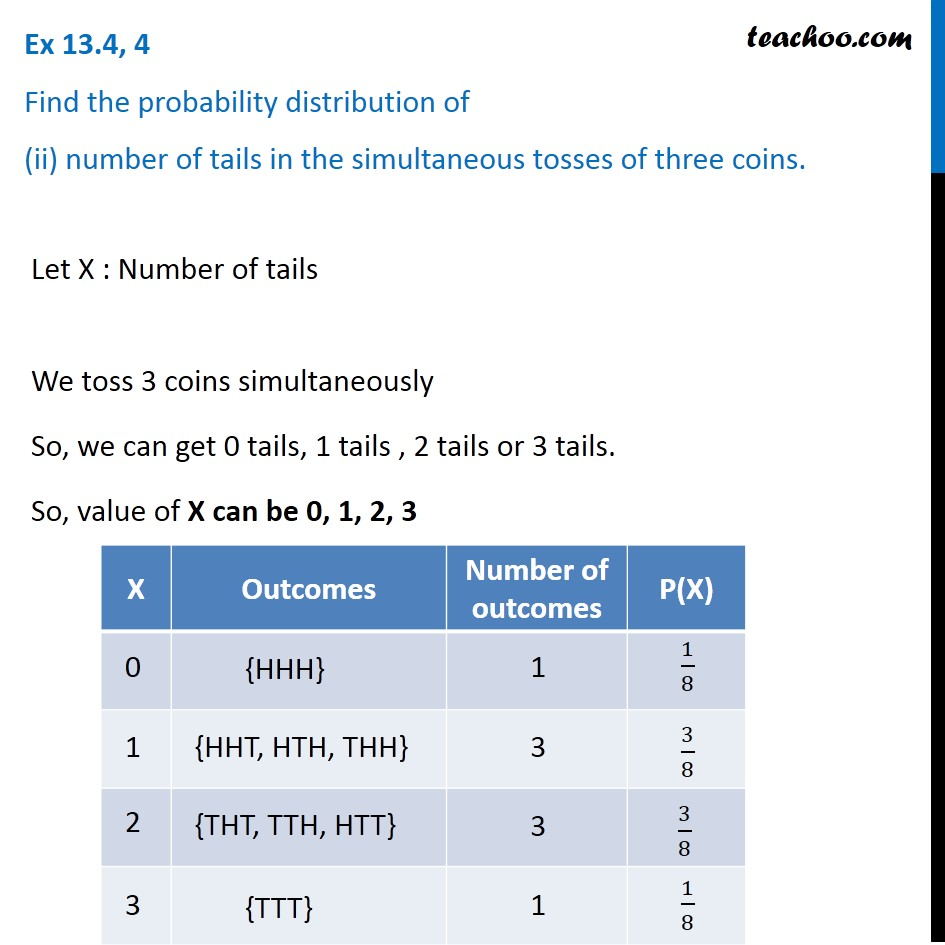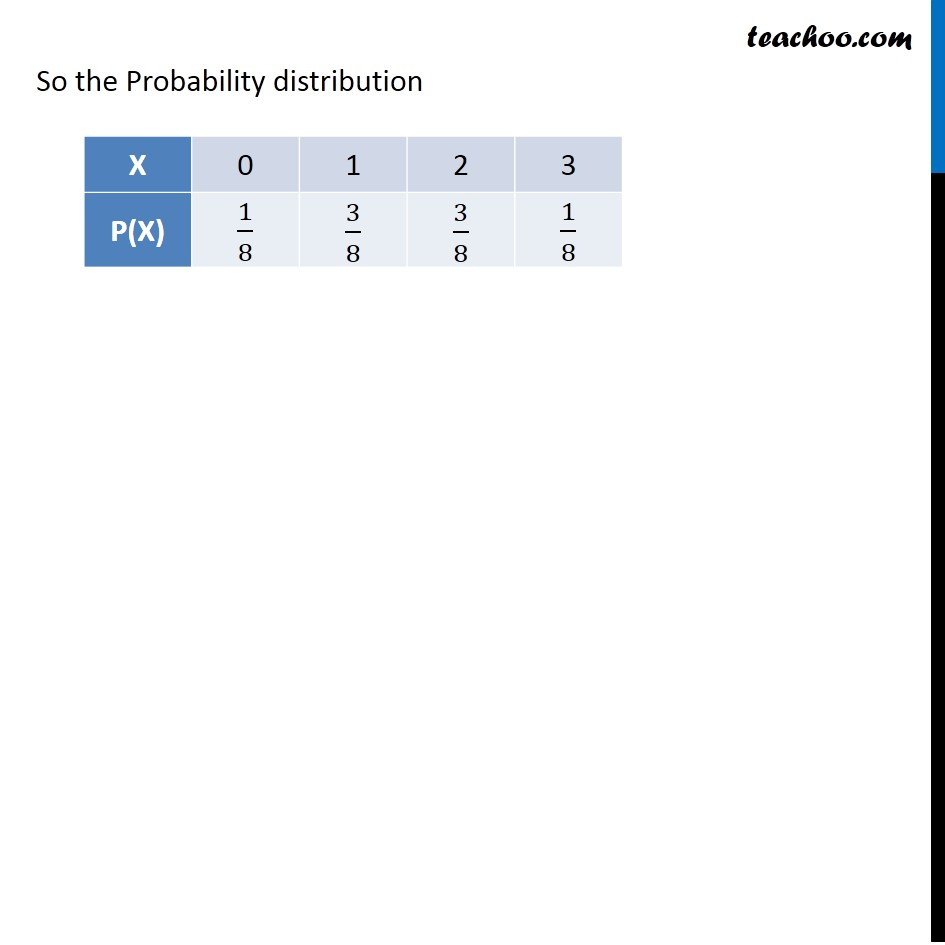Probability Distribution

Chapter 13 Class 12 Probability
Serial order wiseLearn in your speed, with individual attention - Teachoo Maths 1-on-1 Class

### Transcript

Question 4 Find the probability distribution of (ii) number of tails in the simultaneous tosses of three coins. Let X : Number of tails We toss 3 coins simultaaneously So, we can get 0 tails, 1 tails , 2 tails or 3 tails. So, value of X can be 0, 1, 2, 3 So the Probability distribution